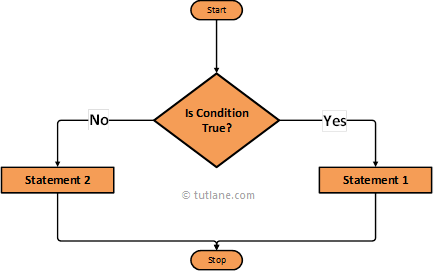# Swift Ternary (?:) Operator

In swift, ternary (?:) operator will perform the same operation whatever is performed by the if-else statement but it’s a shortcut method to perform if-else statement operation.

Now we will see the functionality of the ternary operator in a swift programming language using an algorithm diagram.

## Swift Ternary Operator Flow Diagram

Following is the swift ternary operator flow diagram to represent how the functionality of a ternary operator will work in the swift programming language.If you observe the above swift Ternary operator flow diagram first it will evaluates the given condition is TRUE or FALSE. If given condition is TRUE then it will execute the code block within if statement. In case the given condition is FALSE then it will execute else condition code block and reach the end of the statement.

## Syntax of Swift Ternary Operator

Following is the syntax of a ternary operator in the swift programming language.

condition ? true_expression : false_expression

If you observe above swift ternary operator syntax if given condition is TRUE then it will return first true_expression value otherwise it will return false_expression value.

Now we will see how to use ternary operator in a swift programming language with examples.

## Swift Ternary Operator (?:) Example

Following is the simple example using the ternary operator in swift to check the condition before executing the expressions within the condition.

let x = 10

let y = 20

let result = (x>y) ? ("x is greater than y") : ("y is greater than x")

print(result)

If you observe above swift ternary operator example we are checking the condition that whether “x” value greater than “y” or not based on that we are returning value.

Following is the result of the above swift ternary operator example returned by playground.

y is greater than x

This is how we can use a ternary operator in a swift programming language based on our requirements.